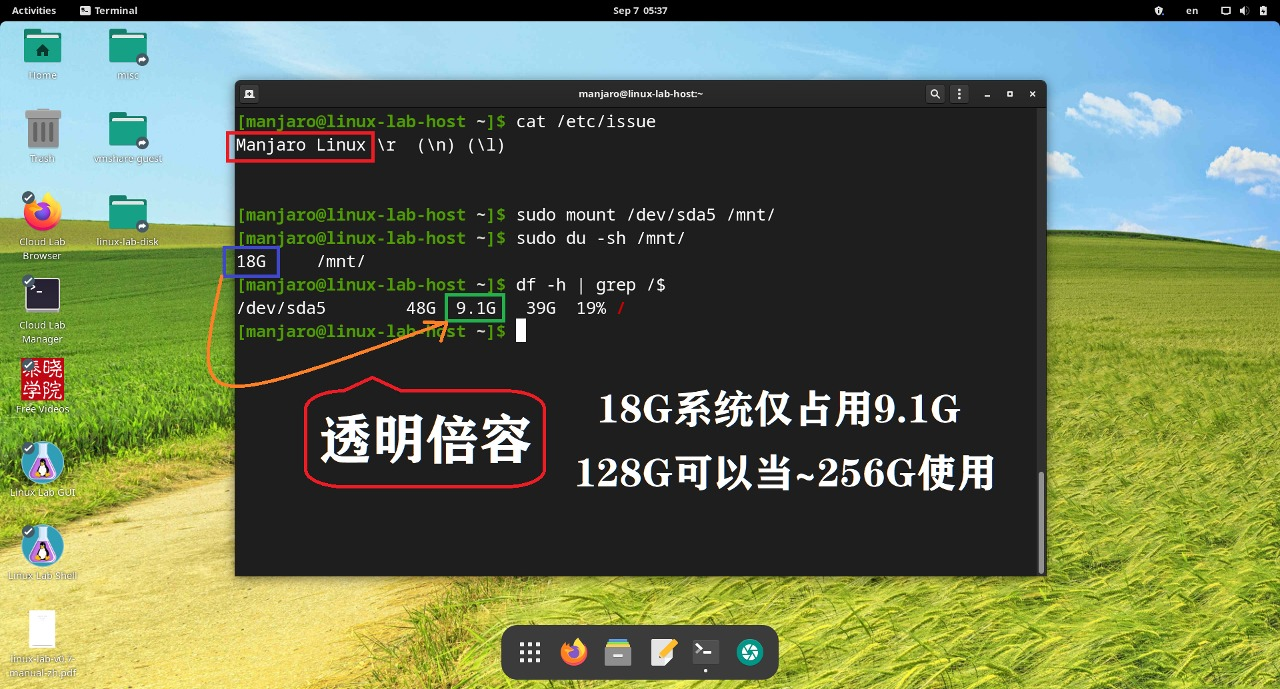# Linux 系统调用

by Pingbo Wen of TinyLab.org 2013/09/12

## 一、用户态

``````execve("/usr/bin/whoami", ["whoami"], [/* 65 vars */]) = 0
brk(0)                                  = 0x100f000
access("/etc/ld.so.nohwcap", F_OK)      = -1 ENOENT (No such file or directory)
mmap(NULL, 8192, PROT_READ|PROT_WRITE, MAP_PRIVATE|MAP_ANONYMOUS, -1, 0) = 0x7f8398cb2000
...
open("/usr/lib/locale/locale-archive", O_RDONLY|O_CLOEXEC) = 3
fstat(3, {st_mode=S_IFREG|0644, st_size=7212544, ...}) = 0
mmap(NULL, 7212544, PROT_READ, MAP_PRIVATE, 3, 0) = 0x7f8397ff2000
close(3)                                = 0
geteuid()                               = 1000
...
open("/etc/passwd", O_RDONLY|O_CLOEXEC) = 3
lseek(3, 0, SEEK_CUR)                   = 0
...
``````

``````#include <sys/types.h>

int main(void)
{
printf("current uid: %dn", geteuid());
return 0;
}
``````

``````#file: dfunc

########################
# Global function
########################
error()
{
echo "\$1"
exit 1
}

show_usage()
{
echo "This is a script to disassemble specified function in a shared library."
echo "Author: WEN Pingbo wengpingbo@gmail.com"
echo "Date: 2013/09/11"
echo "Usage: ./dfunc [-f function name] [-l library path] [-c shift number]"
echo "Note: sometimes, you should using -c to disassemble more bytes because of alignments."
}

########################
# Global function
########################

# default value
func_name="fexecve"
target="/lib/x86_64-linux-gnu/libc-2.15.so"
caliberation="0"

if [ -n "\$1" ] && [ "\$1" == "-h" ];then
show_usage
exit 0
fi

# parse the argument
arg_ok="0"
while [ -n "\$1" ]
do
if [ "\$1" == "-f" ];then
shift
arg_ok="1"
if [ -n "\$1" ];then
func_name="\$1"
shift
fi
fi

if [ "\$1" == "-l" ];then
shift
arg_ok="1"
if [ -n "\$1" ];then
target="\$1"
shift
fi
fi

if [ "\$1" == "-c" ];then
shift
arg_ok="1"
if [ -n "\$1" ];then
caliberation="\$1"
shift
fi
fi

[ "\$arg_ok" == "0" ] && shift
arg_ok="0"
done

offset=`readelf -s "\$target" | grep " "\$func_name"" | awk '{print \$3}'`

offset=\$((\$offset + \$caliberation))

# convert dec to hex
offset=`echo "obase=16; ibase=10; "\$offset"" | bc`

begin=`nm -D "\$target" | grep " "\$func_name"\$" | awk '{print \$1}'`
end=`echo "obase=16; ibase=16; \${begin^^} + \${offset^^}" | bc`

``````

``````syscall
retq
``````

syscall 是什么指令？这里的 syscall 指令就是在 x86 架构下，专门为系统调用准备的指令（ SYSCALL/SYSENTER and SYSRET/SYSEXIT ）。

``````getegid     -   getegid     Ei: __getegid   getegid
geteuid     -   geteuid     Ei: __geteuid   geteuid
getpgid     -   getpgid     i:i __getpgid   getpgid
``````

``````#include <unistd.h>
#include <sys/syscall.h>   /* For SYS_xxx definitions */

int main(void)
{
printf("current uid: %dn", syscall(107));
return 0;
}
``````

``````section .text
global _start

_start:
mov rax,107
syscall ;invoke geteuid()
mov rdi,0 ;return value
mov rax,60 ; _exit syscall
syscall
``````

``````ld -s -o test test.o
strace ./test
``````

``````geteuid()                               = 1000
_exit(0)                                = ?
``````

## 二、内核态

``````106 common  setgid          sys_setgid
107 common  geteuid         sys_geteuid
108 common  getegid         sys_getegid
109 common  setpgid         sys_setpgid
110 common  getppid         sys_getppid
``````

``````{
/* Only we change this so SMP safe */
return from_kuid_munged(current_user_ns(), current_euid());
}
``````

``````{
char tmp[] = "strings from kernel";
if(copy_to_user(str, tmp, 19))
return -EFAULT;

return 0;
}
``````

``````314 common    mysyscall           sys_mysyscall
``````

``````#include <unistd.h>
#include <sys/syscall.h>   /* For SYS_xxx definitions */
#include <stdio.h>

int main(void)
{
char str;
syscall(314, str);
printf("str: %sn", str);
return 0;
}
``````

## 三、系统调用加速

Linux kernel 中，有 vdso 和 vsyscall 的机制，用来加速特定的系统调用。两者的基本原理都是把一些特定的系统调用放到一个专门的 page 中，然后把这个 page 映射到用户程序空间，这样用户态程序就可以不用切换到内核态就可以调用这些函数。

``````libc.so.6 => /lib/x86_64-linux-gnu/libc.so.6 (0x00007f01864b2000)
/lib64/ld-linux-x86-64.so.2 (0x00007f0186894000)
``````

``````0060a000-0060b000 r--p 0000a000 08:03 22020229                           /bin/cat
0060b000-0060c000 rw-p 0000b000 08:03 22020229                           /bin/cat
02267000-02288000 rw-p 00000000 00:00 0                                  [heap]
...
7fff31ccc000-7fff31ced000 rw-p 00000000 00:00 0                          [stack]
7fff31df2000-7fff31df3000 r-xp 00000000 00:00 0                          [vdso]
ffffffffff600000-ffffffffff601000 r-xp 00000000 00:00 0                  [vsyscall]
``````

vdso 的具体实现在 arch/x86/vdso 中，其中的 vdso.lds.S 就定义了具体加速的系统调用。你甚至可以往 vdso 添加自定义的函数，具体添加方法见这里